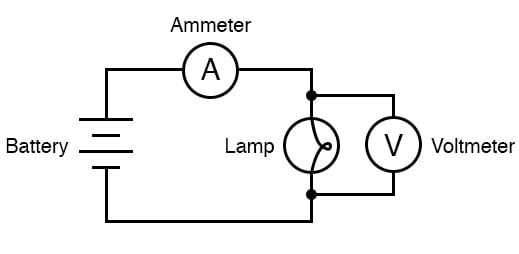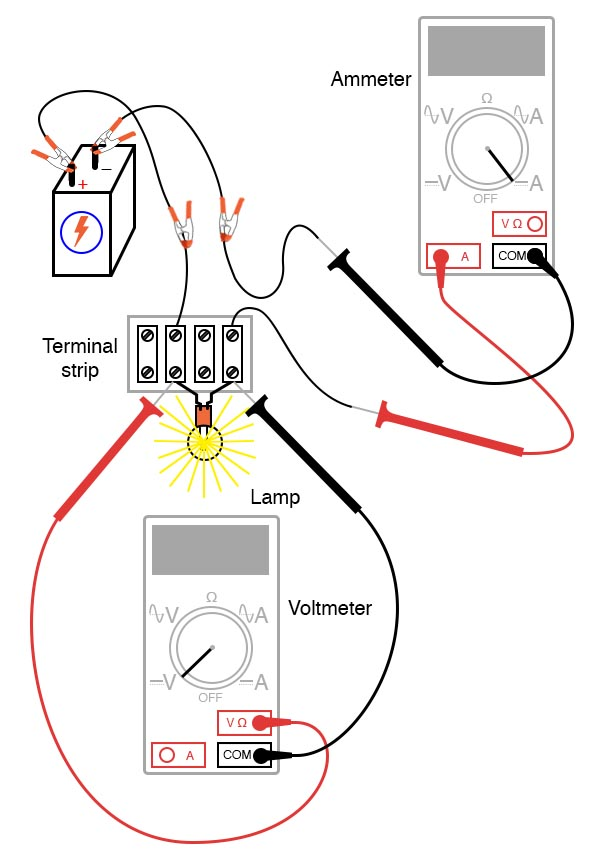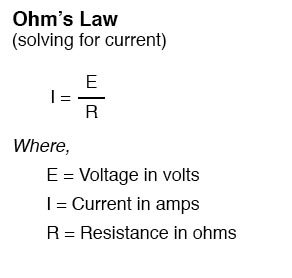# Nonlinear Resistance

## Chapter 2 - Basic Concepts and Test Equipment

PARTS AND MATERIALS

• Calculator (or pencil and paper for doing arithmetic)
• 6-volt battery
• Low-voltage incandescent lamp (Radio Shack catalog # 272-1130 or equivalent)

CROSS-REFERENCES

Lessons In Electric Circuits, Volume 1, chapter 2: “Ohm’s Law”

LEARNING OBJECTIVES

• Voltmeter use
• Ammeter use
• Ohmmeter use
• Use of Ohm’s Law
• Realization that some resistances are unstable!
• Scientific method

SCHEMATIC DIAGRAMILLUSTRATIONINSTRUCTIONS

Measure the resistance of the lamp with your multimeter. This resistance figure is due to the thin metal “filament” inside the lamp.

It has substantially more resistance than a jumper wire, but less than any of the resistors from the last experiment. Record this resistance value for future use. Build a one-battery, one-lamp circuit.

Set your multimeter to the appropriate voltage range and measure voltage across the lamp as it is energized (lit). Record this voltage value along with the resistance value previously measured.

Set your multimeter to the highest current range available. Break the circuit and connect the ammeter within that break, so it becomes a part of the circuit, in series with the battery and lamp.

Select the best current range: whichever one gives the strongest meter indication without over-ranging the meter. If your multimeter is autoranging, of course, you need not bother with setting ranges.

Record this current value along with the resistance and voltage values previously recorded. Taking the measured figures for voltage and resistance, use the Ohm’s Law equation to calculate circuit current. Compare this calculated figure with the measured figure for circuit current:What you should find is a marked difference between the measured current and calculated current: the calculated figure is much greater. Why is this? To make things more interesting, try measuring the lamp’s resistance again, this time using a different model of meter.

You will need to disconnect the lamp from the battery circuit in order to obtain a resistance reading because voltages outside of the meter interfere with resistance measurement. This is a general rule that should be remembered: measure resistance only on an unpowered component!

Using a different ohmmeter, the lamp will probably register as a different value of resistance. Usually, analog meters give higher lamp resistance readings than digital meters.

This behavior is very different from that of the resistors in the last experiment. Why? What factor(s) might influence the resistance of the lamp filament, and how might those factors be different between conditions of lit and unlit, or between resistance measurements taken with different types of meters?

This problem is a good test case for the application of scientific methods. Once you’ve thought of a possible reason for the lamp’s resistance changing between lit and unlit conditions, try to duplicate that caused by some other means.

For example, if you think the lamp resistance might change as it is exposed to light (its own light, when lit) and that this accounts for the difference between the measured and calculated circuit currents, try exposing the lamp to an external source of light while measuring its resistance. If you measure substantial resistance change as a result of light exposure, then your hypothesis has some evidential support.

If not, then your hypothesis has been falsified, and another cause must be responsible for the change in circuit current.

• Share
Published under the terms and conditions of the Design Science License